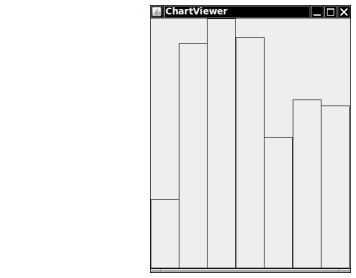Write a class BarChart with methods

public void draw(Graphics2D g2)

that displays a chart of the added values. You may assume that all added values are positive. Stretch the bars so that they fill the entire area of the screen. You must figure out the maximum of the values, and then scale each bar.

Here is a sample program output:Complete the following file:

### BarChart.java

Use the following files:

BarChartComponent.java

```import javax.swing.JComponent;
import java.awt.Graphics;
import java.awt.Graphics2D;

public class BarChartComponent extends JComponent
{
public void paintComponent(Graphics g)
{
Graphics2D g2 = (Graphics2D) g;
BarChart c = new BarChart(getWidth(), getHeight());
c.draw(g2);
}
}
```

ChartViewer.java

```import javax.swing.*;

/**
Displays a bar chart.
*/
public class ChartViewer
{
public static void main(String[] args)
{
JFrame frame = new JFrame();

final int FRAME_WIDTH = 300;
final int FRAME_HEIGHT = 400;

frame.setSize(FRAME_WIDTH, FRAME_HEIGHT);
frame.setTitle("ChartViewer");
frame.setDefaultCloseOperation(JFrame.EXIT_ON_CLOSE);

BarChartComponent component = new BarChartComponent();